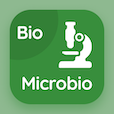Computer Science Courses Online

C++ Practice Tests

C++ Online Tests

The Book Permutation Function Multiple Choice Questions (MCQ Quiz) with answers, Permutation Function MCQ Quiz PDF download to study online c++ degree courses. Study Functions in C++ Multiple Choice Questions and Answers (MCQs), Permutation Function quiz answers PDF for 2 year computer science degree. The e-Book Permutation Function MCQ App Download: inline functions, header files, standard c library functions test prep for top computer science schools in the world.

The MCQ: Which of the following function returns no value? PDF, "Permutation Function" App Download (Free) with printdate ( ), sqrt ( ), log ( ), and cos ( ) choices for 2 year computer science degree. Practice permutation function quiz questions, download Google eBook (Free Sample) for CS major.

## C++ MCQs: Permutation Function Quiz Questions

MCQ: Which of the following function returns no value?

A) printDate ( )
B) sqrt ( )
C) log ( )
D) cos ( )

MCQ: A function that need no return value, is called

A) Infinite function
B) Finite function
C) Void function
D) All of them

MCQ: A permutation is an arrangement of elements taken form

A) Infinite set
B) Finite set
C) Characters
D) Strings

MCQ: Void function is also called as

A) Procedure
B) Subroutine
C) Terminator
D) Both A and B

### Practice Tests: C++ Exam Prep

Download C++ Quiz App, HCI MCQ App, and Microbiology MCQs App to install for Android & iOS devices. These Apps include complete analytics of real time attempts with interactive assessments. Download Play Store & App Store Apps & Enjoy 100% functionality with subscriptions!